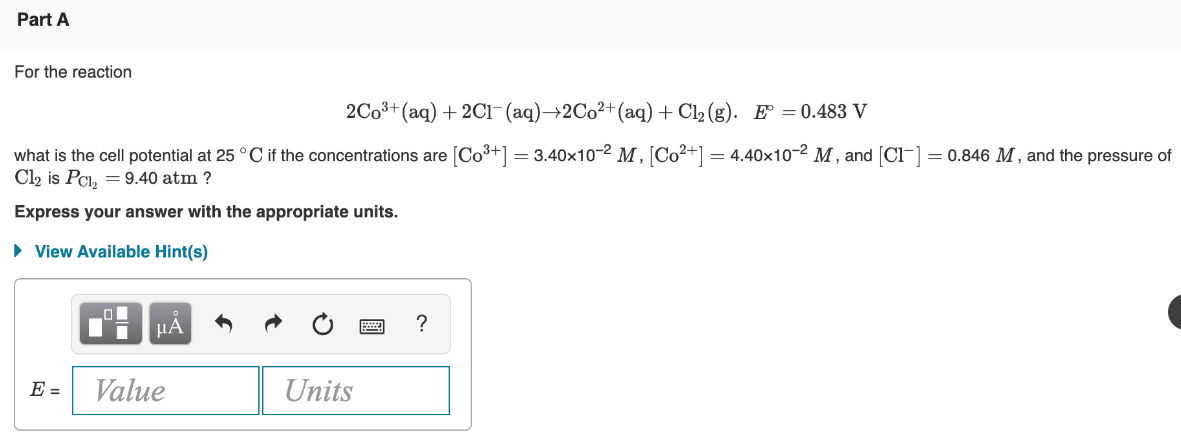# For the reaction °= 2Co^3+ (aq) + 2Cl^-(aq) → 2Co^2+ (aq) + Cl2(g) • E° = 0.483 V what is the cell potential at 25 °C if the concentrations are [Co^3+] = 3.40x10^-2 M, [Co^2+] = 4.40x10^-2 M, and [Cl^-] = 0.846 M, and the pressure of Cl2 is PCl2 = 9.40 atm? Express your answer with the appropriate units.